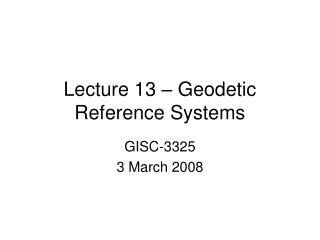DownloadDownload PresentationLecture 13 – Geodetic Reference Systems

# Lecture 13 – Geodetic Reference Systems

Download Presentation## Lecture 13 – Geodetic Reference Systems

- - - - - - - - - - - - - - - - - - - - - - - - - - - E N D - - - - - - - - - - - - - - - - - - - - - - - - - - -
##### Presentation Transcript

1. Lecture 13 – Geodetic Reference Systems GISC-3325 3 March 2008

2. Update • Exam scheduled for 13 March 2008 • Covers lectures, labs, homework, required readings and chapters 5-7. • Required reading: • Burkholder 3D Cogo article

3. 3D Coordinate Systems • Geodetic (Curvilinear) Coordinates • Latitude, longitude and ellipsoid height • Right-handed, earth-centered earth-fixed, positive east • Geocentric (Cartesian) Coordinates • X, Y, Z • Likewise, ECEF, right-handed, • Orthogonal

4. GPS vectors Difference in geocentric coordinates. Both difference in geocentric coordinates and changes in local geodetic horizon coordinates.

5. Local Geodetic Horizon (LGH) • ECEF, right-handed, orthogonal, 3-D • Origin at any point specified • N in meridian plane oriented twd N pole • U normal to ellipsoid at origin • E perpendicular to meridian plane

6. LGH

7. Geodetic azimuth. Mark-to-mark slant range. Vertical or zenith angle.

8. Geodetic to Geocentric Coordinate Conversions

9. Geocentric to Geodetic

10. Geocentric to Geodetic • We use ellipsoid parameters (a, f-1) • Calculate preliminary values (set: h = 0) • Lat1 = atan( (Z / sqrt(x2+y2))*(1/(1-e2)) • N1 = a / sqrt(1-e2*sin(Lat1)2) • h1 = (sqrt(x2+y2)/cos(Lat1))-N1 • We iterate using these starting values • We stop iterating when the shift in ellipsoid height is within our accuracy goal.

11. 2D-Coordinate Transformations • Given • x = r * cos(γ) • y = r * sin(γ) • Rotate coordinate system byΘ • x’ = r * cos(γ – Θ) • y’ = r * sin(γ – Θ) • Use trig identities to solve recollect • cos(γ – Θ) = cos γcos Θ+sin Θsin γ • sin(γ – Θ) = sin γ cos Θ – cos γ sin Θ

12. Translation • If we shift the origin we can update coordinates by merely adding/subtracting shift from matching coordinate. • x’ = x – tx • y’ = y - ty

13. Scale change • We can scale coordinates to account for issues like m to ft. • x’ = s * x • y’ = s * y

14. Four-parameter transformation • Combines rotations, translations and scale in one operation. • x’ = s*(x*cos Θ+y*sin Θ) + tx • y’ = s*(-x*sin Θ+y*cos Θ)+ty • Matrix form is simpler

15. Three-Dimensional Transformation • 7-parameters • scale • rotations along X,Y,Z axes • translations in X,Y,Z

16. Euler matrices and 7-parameter Matrix D for rotation on Z axis Matrix C for rotation of Y axis Matrix B for rotation of X axis

17. Euler matrices

18. Application of 7-para transf